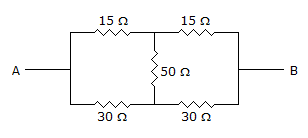# Electronics and Communication Engineering - Exam Questions Papers

11.

Consider the circuit shown below of 2 : 1 MUX is given by the function g = ac + bc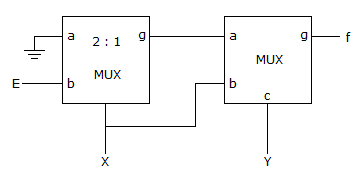Then f is

 A. X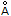X B. XY + E X Y C. XE + XY D. None of these

Explanation:

g = ac + bc

min c = 0, g = b and c = 1, g = a

So f = y + yay x + yg ...(i)

g = x b + x.a = x E + X.ox E

from (i) f = y x + y x E.

12.

For the given phase lead network, the maximum possible phase lead is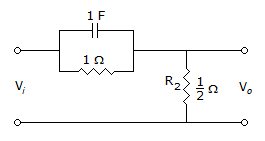A. sin-1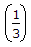B. 30° C. 45° D. 60°

Explanation:

The maximum phase lead is given by,

sin φm =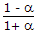Where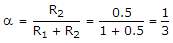Hence sin φm =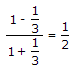Hence φm = 30°.

13.

Given (224)r =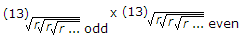The value of the radix 'r' is :

 A. 5 B. 6 C. 7 D. 10

Explanation:

(224)r == (13)r x (13)r

2r2 + 2r + 4 = (r + 3) (r + 3) = r2 + 6r + 9

r2 - 4r - 5 = 0

(r - 5) (r + 1) = 0

r = - 1 or r = 5

Negative base is not possible. Therefore base of the equation is 5.

14.

Which factor determines whether the medium is free space, lossless dielectric, lossy dielectric or good conductor?

 A. Attenuation constant B. Constitutive parameters (σ, μ, ε) C. Loss tangent D. Reflection coefficient

Explanation:

Loss tangent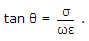(i) for lossless dielectric, tan θ ≤ 1

(ii) for good conductor, tan θ >> 1

(iii) for lossy dielectric, tan θ = 1.

15.

Find RAB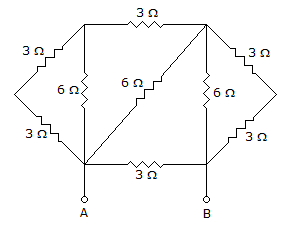A. 2 Ω B. 1.5 Ω C. 3 Ω D. None of the above

Explanation:

Reduced Diagram :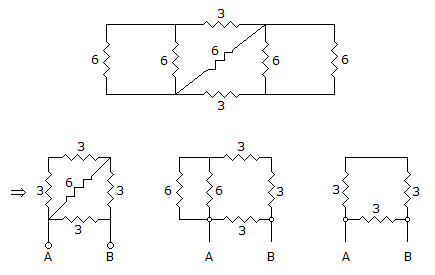RAB = 3 || 6 = 2